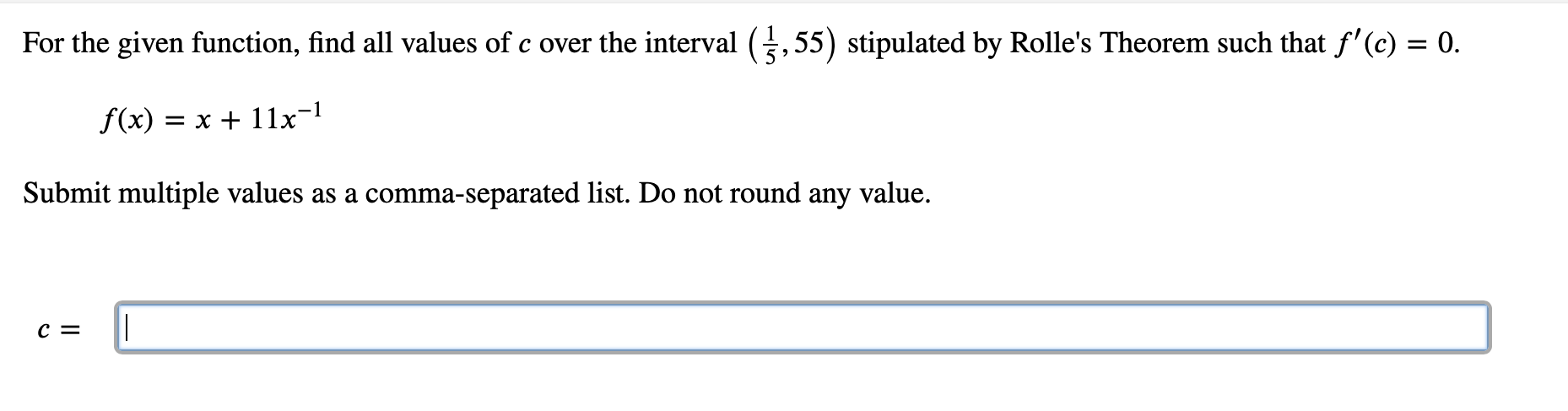# For the given function, find all values of c over the interval (,55) stipulated by Rolle's Theorem such that f'(c) = 0. f(x) = x + 11x=l Submit multiple values as a comma-separated list. Do not round any value. c =

Questionhelp_outlineImage TranscriptioncloseFor the given function, find all values of c over the interval (,55) stipulated by Rolle's Theorem such that f'(c) = 0. f(x) = x + 11x=l Submit multiple values as a comma-separated list. Do not round any value. c = fullscreen

### Want to see this answer and more?

Experts are waiting 24/7 to provide step-by-step solutions in as fast as 30 minutes!*

*Response times may vary by subject and question complexity. Median response time is 34 minutes for paid subscribers and may be longer for promotional offers.
Tagged in
MathCalculus

### Derivative